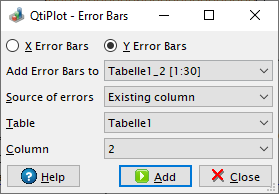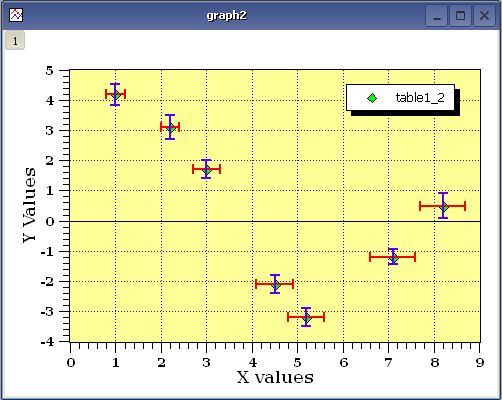This dialog is activated by selecting the Add Error Bars... command from the Graph menu.

This command is used to plot X and/or Y error bars at each data point.

Note that clicking on the "Close" button will not add any error bars to the plot. You must set up the parameters for the error bars first and then click on the "Add" button. If you click on "Close" without clicking "Add", there will be no error bars drawn.

Figure 5-9. The Error bars dialog.There are five ways to specify the sizes of the bars:

1) From a column of the table:

In this case, the values in the selected column are used to compute the error bars. Specifically, if V is the value of a data point, and E the value from the same row of the errorbar column, the size of the corresponding bar will be V-E to V+E.

2) A percentage of the values:

This command adds a new column to the table associated with the plot and fills it with result of calculating V*E/100 for each row in the table, where V is the plotted value and E is the selected percentage. This column is then used to plot the error bars in exactly the same manner as in the previous case. The new column can subsequently be edited like any other column. Any changes will be reflected in the size of the corresponding error bars.

3) The standard deviation of the values:

This command first calculates the standard deviation of the plotted values. This has meaning only if the data are centered around an average value. As with the previous option, a new column will be created in the active table and each row will be filled with the computed standard deviation. The program does not know and cannot compute individual standard deviations at each point. If this is required, you will need to independently compute these values from multiple samples taken at each x or y value.

4) The standard error of the values:

This command first calculates the standard error of the plotted data values. A new column will be created in the active table and each row will be filled with the computed standard error.

5) The square root of the values:

This command first calculates the square root of the plotted data values. A new column will be created in the active table and each row will be filled with the computed square root.

The default style of the error bars (color, line width, cap length, etc...) can be customized via the Error Bars tab of the Preferences dialog.

If you need to display asymmetric error bars you must define two sets of error bars for the same curve: one for the upper limits (with disabled minus side) and one for the lower limits (with disabled plus side).

Figure 5-10. Example of a plot with both X and Y Error Bars.